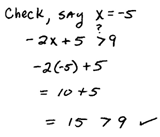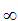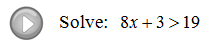## Pages

### Introduction to Inequalities and Interval Notation

All of the steps that we have learned for solving linear equations are the same for solving linear inequalities except one.  We may add or subtract any real number to both sides of an inequality and we may multiply or divide both sides by any positive real number.

The only new rule comes from multiplying or dividing by a negative number.So whenever we divide or multiply by a negative number we must reverse the inequality. It is easy to forget to do this so take special care to watch out for negative coefficients.Notice that we obtain infinitely many solutions for these linear inequalities.  Because of this we have to present our solution set in some way other than a big list.  The two most common ways to express solutions to an inequality are by graphing them on a number line and interval notation.

Note: We use the following symbol to denote infinity:Tip: Always use round parentheses and open dots for inequalities without the equal and always use square brackets and closed dots for inequalities with the equal.